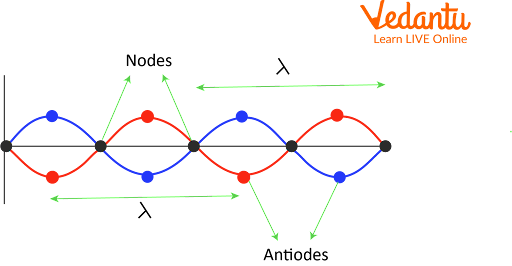Courses
Courses for Kids
Free study material
Free LIVE classes
More

# Difference Between Node and AntinodeLIVE
Join Vedantu’s FREE Mastercalss

## Stepping Into the Realm of Oscillations and Waves: An Introduction to Wave Motion

A wave is an energetic disturbance in a medium that doesn't include any net particle motion. Elastic deformation, a change in pressure, an electric or magnetic intensity, an electric potential, or a change in temperature are some of the possible manifestations. Standing wave is a type of wave which gives us an idea about what is a node and an antinode.

Last updated date: 22nd Sep 2023
Total views: 6.6k
Views today: 0.06k

## What is a Standing Wave: Understanding the Mechanism Behind Standing Waves

Different from a travelling wave is a standing wave. A standing wave forms where each point oscillates about the wave's axes. While the points of a specific phase remain at a constant location across time, these nearby points continue to be in phase. Since all nearby points are in phase, no energy is transferred between them.

The superposition of two travelling waves of the same frequency but going in opposite directions results in the formation of standing wave. To guarantee the same frequency, the travelling wave and its reflection are employed. Let's discuss the nodes and antinodes of a standing wave.

## Characteristics of Stationary Waves:

The primary traits or qualities of standing waves are as follows:

• Standing wave particles in adjacent segments vibrate in the opposite phase from those in the same division, who vibrate in the same phase.

• Particle vibration does not involve any energy transfer or exchange.

• Half of the distance between one node and its adjacent antinode is the difference between two successive nodes or antinodes.

## Antinode and Node of the Standing Wave:

The spot on a standing wave known as a node stays in place over time at a fixed position. Due to two waves interfering destructively, this is the cause. The wave where particle vibration is at its strongest is called the antinode wave.

The standing wave therefore has its largest amplitude at the antinode and its minimum amplitude at the node. Any standing wave's endpoints are called nodes for this reason.Nodes and Antinodes on a Standing Wave

## Formula for Finding Position of Nodes and Antinodes on a Standing Wave:

### For Antinodes:

$2a\sin \left (kx\right )=1$

$sinkx = sin \left ( \left ( n + 1/2 \right )\pi/2 \right )$

$kx = \left (n + 1/2 \right )\pi/2$

$x = \left ( n + 1/2 \right )\lambda/2$

For n=0,1,2,...

Where, a= amplitude of the wave

k= wave propagation constant

\lambda= wavelength

### For Nodes:

$2a\sin \left (kx\right )=0$

$\sin \left (kx\right )=0$

$\sin \left (kx\right )= \sin\left ( n\pi \right )$

$kx=n\pi$

$x=n\lambda$

For n=0,1,2,...

Where a= amplitude of the wave

k= wave constant

## Differentiate Between Node and Antinode of a Standing Wave:

 S.No Category Nodes Antinodes Significance They signify the position of zero amplitude. They signify the position of the highest amplitude. Location Consecutive nodes are separated by half a wavelength. Located exactly at the midpoint of two consecutive nodes. Velocity of particles Velocity of particles is zero at the nodes Velocity of the particles at antinodes is maximum in a standing wave Change in pressure Change in pressure experienced at nodes tends to be maximum. Change in pressure experienced at antinodes tends to be at minimum. Formula for positions on wave $x=n\lambda$For n=0,1,2,...And,  \lambda= wavelength $x = \left ( n + 1/2 \right )\lambda/2$For n=0,1,2,...Where,  \lambda= wavelength 6. node and antinode examples Ends of a vibrating guitar chord are an example of nodes Antinode of the same guitar chord lies between the two consecutive nodes.

## Summary

Standing waves, nodes, and antinodes are simple to comprehend and can be better understood through node and antinode examples. The standing wave's node and antinode are simple to examine and distinguish from one another. Numerous practical applications, such as those in woodwind and string instruments, are available for this study of waves. The readers will find it simple to comprehend the standing wave behaviour once they have gone over the fundamentals of nodes and antinodes along with how to differentiate between node and antinode.

## FAQs on Difference Between Node and Antinode

1. What is node and antinode?

In standing waves, the nodes are the locations where the vibration's amplitude is zero, while the antinodes are the locations where the vibration's amplitude is greatest. Antinodes oppose one another because they are found in the space between nodes. Nodes are the points of minima whereas antinodes are the points of maxima in a standing wave.

2. What are some examples of Standing waves in real life?

The strings on a guitar, or any other string instrument, are a well-known example of standing waves in the real world. In these instruments, a plucked string produces a certain frequency based on the string's length, tautness, and density. Nodes lie at the ends of the strings whereas antinodes lie between the nodes.

3. What are the applications of standing waves in the field of medicine?

The combination of two waves with the same frequency and amplitude but opposite motion results in standing waves. Some of the applications of standing waves in the field of medicine include utilization of stationary waves in the absorption of X-Rays, CT Scanners, Diagnostic X-Rays, studying Electrocardiograph, Fibre optics and endoscopy.